# Use the First Derivative Test to find the relative extrema of the function, if they exist....

Use the First Derivative Test to find the relative extrema of the function, if they exist. f(x) = x^4 - 2x^2 + 5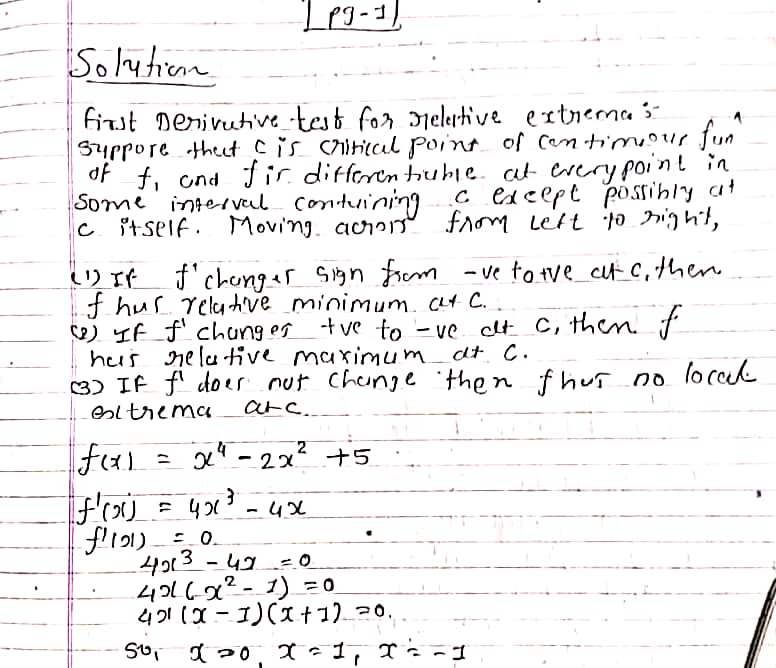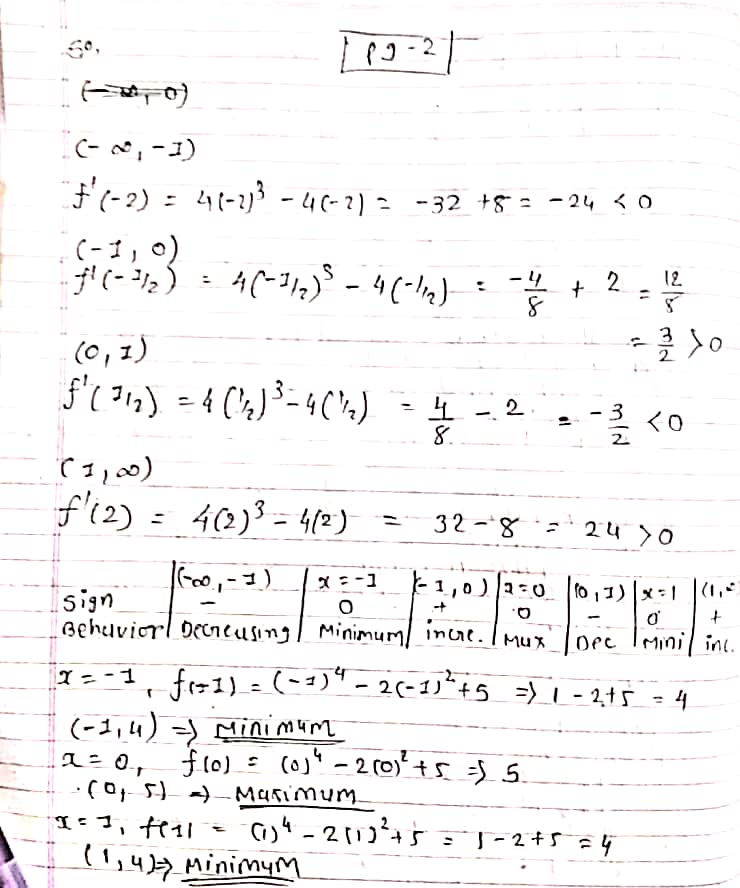#### Earn Coin

Coins can be redeemed for fabulous gifts.

Similar Homework Help Questions
• ### Find all relative extrema of the function. Use the Second-Derivative Test when applicable. (If an answer...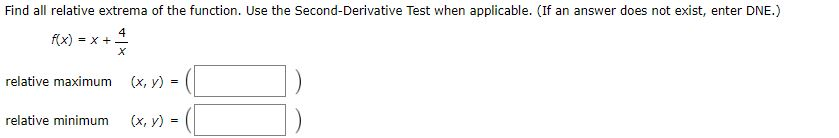Find all relative extrema of the function. Use the Second-Derivative Test when applicable. (If an answer does not exist, enter DNE.) f(x) = x+ 4 relative maximum (x, y) = relative minimum (x, y)

• ### Find all relative extrema of the function. Use the Second-Derivative Test when applicable. (If an answer...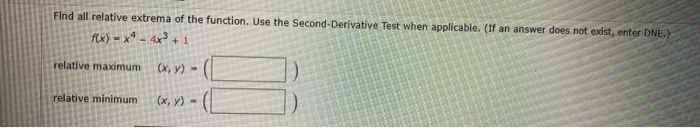Find all relative extrema of the function. Use the Second-Derivative Test when applicable. (If an answer does not exist, enter DNE.) f(x) = x4 - 4x3 + 1 relative maximum (x,y) - relative minimum (x, y)

• ### Find all relative extrema of the function. Use the Second Derivative Test where applicable. (If an...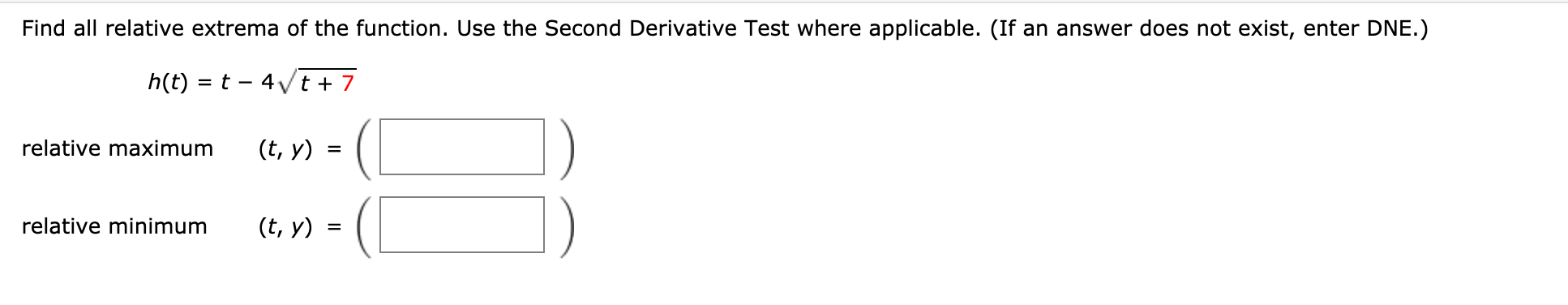Find all relative extrema of the function. Use the Second Derivative Test where applicable. (If an answer does not exist, enter DNE.) h(t) = t - 4vt + 7 relative maximum (t, y) relative minimum (t, y)

• ### 3. Find the relative extrema of f(x)= 2r3 +32- 12r-4, and use the second derivative test...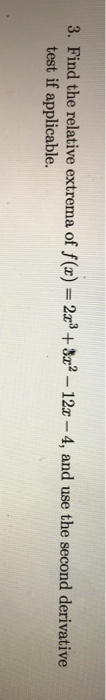3. Find the relative extrema of f(x)= 2r3 +32- 12r-4, and use the second derivative test if applicable. 3. Find the relative extrema of f(x)= 2r3 +32- 12r-4, and use the second derivative test if applicable.

• ### 8. [1/2 Points] DETAILS PREVIOUS ANSWERS LARAPCALC10 3.3.022. Find all relative extrema of the function. Use...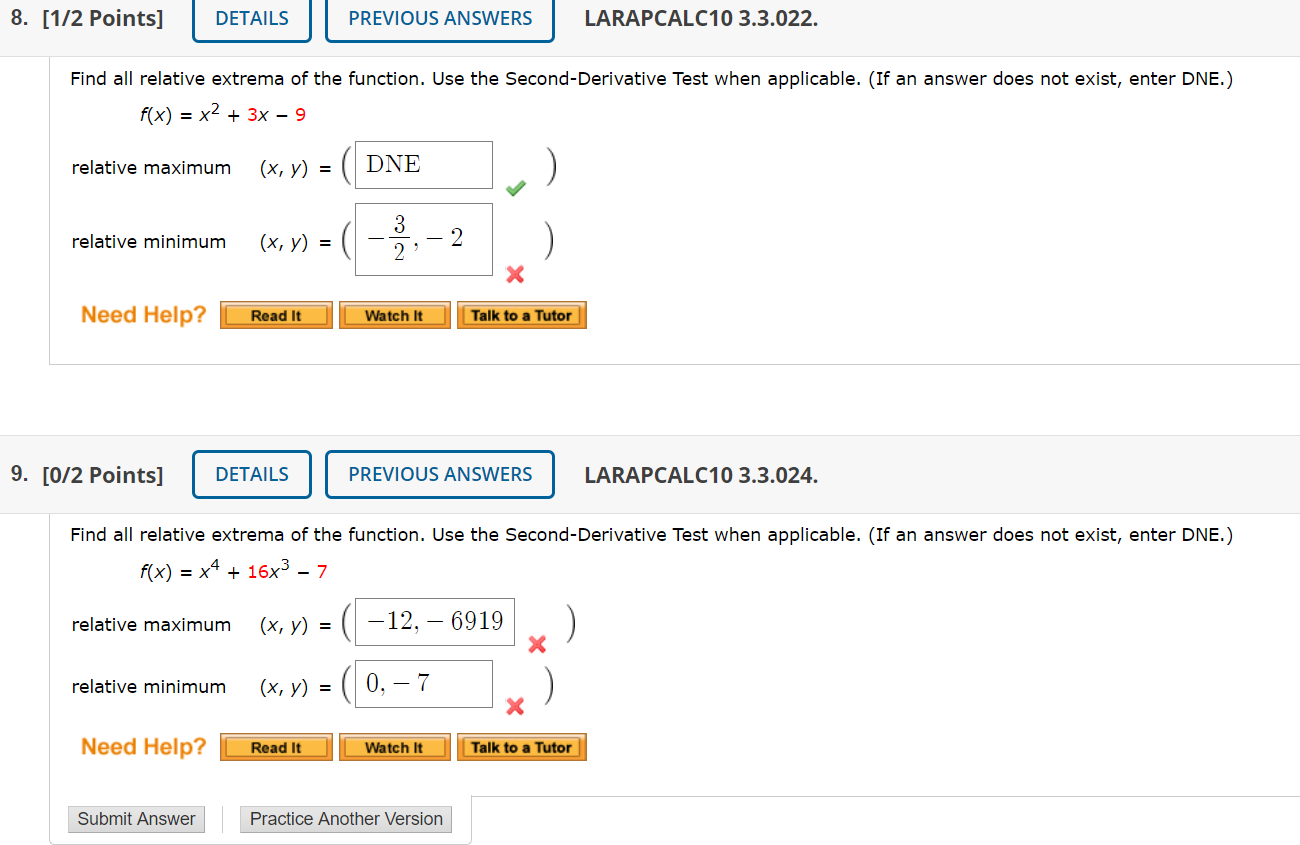8. [1/2 Points] DETAILS PREVIOUS ANSWERS LARAPCALC10 3.3.022. Find all relative extrema of the function. Use the Second-Derivative Test when applicable. (If an answer does not exist, enter DNE.) f(x) = x2 + 3x – 9 relative maximum (x, y) = DNE ) relative minimum (x, y) = 3 2 – 2 x Need Help? Read It Watch It Talk to a Tutor 9. [0/2 Points] DETAILS PREVIOUS ANSWERS LARAPCALC10 3.3.024. Find all relative extrema of the function. Use the...

• ### Find the relative extrema and the points of inflection (if any exist) of the function. Use...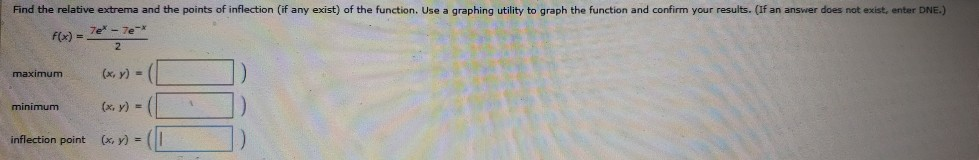Find the relative extrema and the points of inflection (if any exist) of the function. Use a graphing utility to graph the function and confirm your results. (If an answer does not existenter DNE.) F(x) = 7er - 7e- 2 maximum (x, y) minimum (x, y) = 10 inflection point (x, y) = (1

• ### Apply the second derivative test to find the relative extrema of the function f(x)=ln(x2+x+1)

Apply the second derivative test to find the relative extrema of the function f(x)=ln(x2+x+1)

• ### Use the first derivative test to find local extrema Question h(x) = x3 + 32x2 +...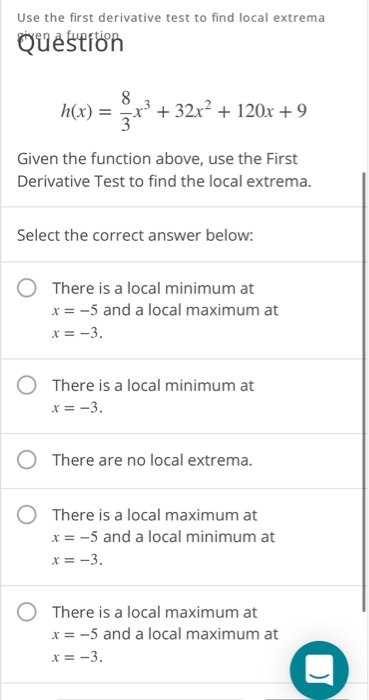Use the first derivative test to find local extrema Question h(x) = x3 + 32x2 + 120x + 9 Given the function above, use the First Derivative Test to find the local extrema. Select the correct answer below: There is a local minimum at x = -5 and a local maximum at x = -3. O There is a local minimum at x = -3. O There are no local extrema. O There is a local maximum at x =...

• ### 2. 3 points TanApCalc9 8.3.019. My Notes Find the critical point of the function. Then use the second derivative test to classify the nature of this point, if possible. (If an answer does not exist,...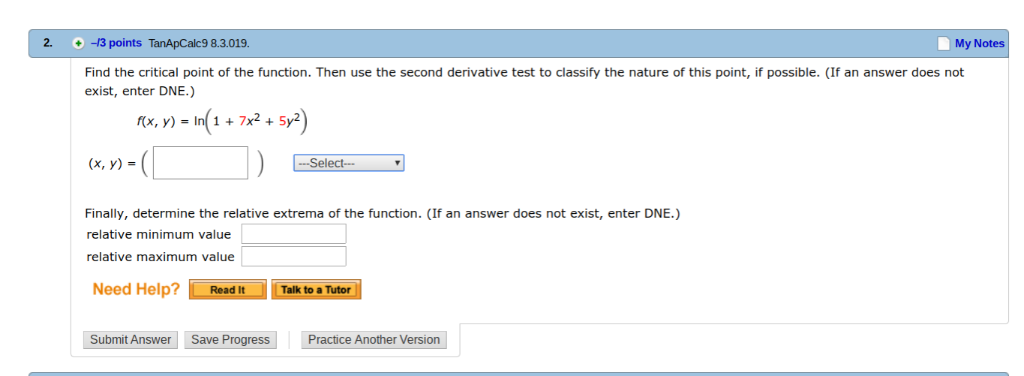2. 3 points TanApCalc9 8.3.019. My Notes Find the critical point of the function. Then use the second derivative test to classify the nature of this point, if possible. (If an answer does not exist, enter DNE.) f(x, y) In 1+7x2 + 5y2 Select (x, y)s Finally, determine the relative extrema of the function. (If an answer does not exist, enter DNE.) relative minimum value relative maximum value Need Help? Read It Talk to a Tutor Submit Answer Save Progress...

• ### Find the critical point of the function. Then use the second derivative test to classify the...

Find the critical point of the function. Then use the second derivative test to classify the nature of this point, if possible. (If an answer does not exist, enter DNE.) f(x, y) = x2 − 4xy + 2y2 + 4x + 8y + 8 critical point (x, y)= classification ---Select--- :relative maximum, relative minimum ,saddle point, inconclusive ,no critical points Finally, determine the relative extrema of the function. (If an answer does not exist, enter DNE.) relative minimum value= relative...